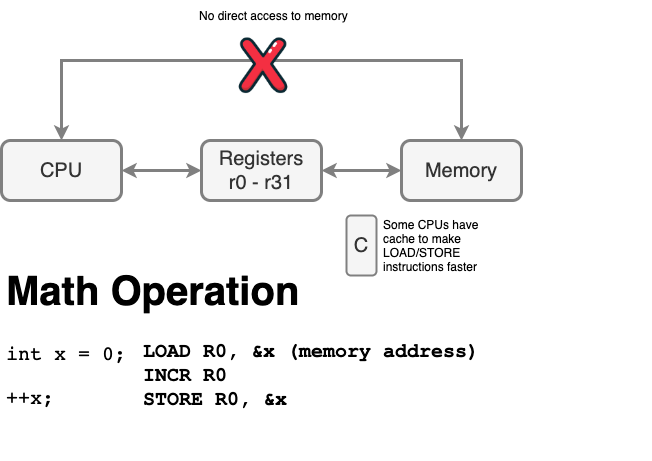# C vs. C++

Typically, C design pattern to create a re-usable module is performed like so:

``````typedef struct {
int target_ms;
int interval_ms;
} timer_t;

void timer_start(timer_t *t, uint32_t interval);

void timer_stop(timer_t *t);

bool timer_expired(timer_t *t);``````

On the other hand, C++ for the same module would be:

``````class timer
{
public:
void start(uint32_t interval);
void stop();
bool expired();

private:
int target_ms;
int interval_ms;
};``````

Code analysis

``````void main(void)
{
/**
* C convention:
*   object_method(&obj)
* Then you pass the object as the first parameter to the methods to "operate on" this object
*/
timer_t t;
timer_start(&t, 1000);
timer_stop (&t);

/**
* C++ convention:
*  obj.method()
*
* C++ automatically passes the object pointer, known as the "this"  pointer to the method
* In reality, the language and the compiler is invoking the methods just like C:
*    timer::start(&obj, 1000);
*/
timer t;
t.start(1000);
t.stop();
}``````

# Volatile Variable``````// volatile
int flag; // global memory is part of "BSS" section, guaranteed to be zero (unless a bug in startup code)

while (1) {
++flag;
}
}

while (1) {
flag = 0;
while (0 == flag) {
;
}

puts("flag has incremented away from value of 0");
}
}
``````

#### Interview Questions

• What is a `volatile` keyword?
• Given a code snippet, why you cannot set a breakpoint?
• Is `const` opposite of volatile?
• What is the point of "`const volatile *variable`" ?
• You as a programmer, cannot write a line of code to modify, but maybe DMA or a peripheral behind you can modify it